Calculation formulas of multilayer inductor with rectangular former - 3.0 out of 5 based on 1 vote
Rating 3.00 (1 Vote)

Calculation formulas of multilayer inductor with rectangular formerFaced with difficulties in the calculation of the multilayer coil on a solenoidal former, we have abandoned the simple empirical Wheeler's formula and went by the way of complication of the inductor model and the calculation algorithm. In the case of a multilayer coil on a rectangular former, which is quite often used, it is obvious that we should go by the same way. This coil can be represented as a set of coaxial rectangular loops and we can calculate the total inductance of the coil as the sum of own and mutual inductances of these loops.

But we can go further and imagine the coil as a set of straight wires. Each turn is a set of four straight segments. Moreover, mutually perpendicular segments do not interact with each other and their mutual inductance is zero. Using the fact that the rectangle is a symmetric figure, we can perform calculations only for two of its sides. Doubling the result of the calculation, we obtain the total inductance of the coil. The following figure shows two parallel wires unequal length for the true understanding of formulas.For such calculations, we need to know the following...

1. The formula of self-inductance of straight wire:where:
• L - inductance [µH]
• l = l1 or l = l2 - length of wire [mm];
• r = d/2 - the radius of the conductor [mm];
2. More commonly known formula which is a simplification of the above, but we do not it use, because less accurate:3. The formula for mutual inductance of two wire segments of unequal length:where:
• M - the value of mutual inductance [µH];
• x1 = l1/2 - half the length of the first conductor [mm];
• x2 = l2/2 - the half-length of the second conductor [mm];
• D - the distance between the centers of the conductors [mm];

The formula is true for conductors, the centers of which lie on the same axis as is the case for our coil.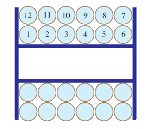The coil is calculated by a numerical method "virtual winding". When you add a new turn, is calculated self-inductance of all its segments, as well as possible mutual induction with all the other segments, taking into account the mutual directions of current in them. In result, we obtain the self-inductance of the multilayer coil. At the same time, we calculate  DC resistance and length of wire required for winding. In addition to the plug-in "multi_rectangular" to the Coil32 program for Windows, which is available for download from the program menu and calculates such inductor, you can use the online calculator of multilayer coil on a rectangular former.

The real loop has rounded corners and does not represent a perfect rectangle. This circumstance, as well as the limited accuracy of the original formulas, do not allow to achieve high precision of the calculation. The error of calculation is about ±5% of the value of the inductance. We mean that the winding is dense, without gaps and interlayer shims. Despite the low accuracy, this numerical algorithm allows the calculation of arbitrary multi-layer coil on a rectangular former without resorting to searching complicated empirical formulae, are always constrained by the geometry of the winding. Using this algorithm, for example, we can easily calculate the inductance of a multi-turn rectangular loop of large diameter.

References:

Сomments from anonymous guests are enabled with moderation.

Coil Winding Machine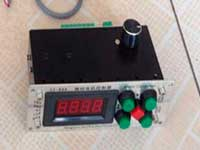US \$187.53
The system consists of individual components including a controller, a stepping motor, a foot switch, power adapter and output shaft. This design makes it very easy for you to integrate the system into your own project.

LC Meter LC200A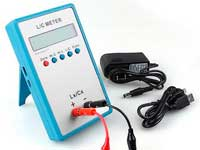US \$37.99
Measuring Capacitance Range:
0.01 pF - 100mF
Measuring Inductance Range:
0.001 uH - 100H
Measurement accuracy:1%.

RF Inductors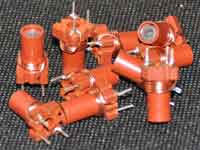US \$6.43
Variable Ferrite Core Inductor coil set 3.5Turns 25-100MHZ

Auto Ranging LCR Meter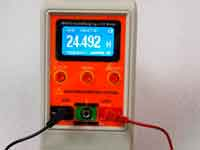US \$63.07
Meter can measure 0.00pF to 100.00mF capacitance, 0.000uH to 100.00H inductance and 0.00? to 10.000M? resistance. Very suitable for small value capacitor and inductor measurement.